# Chapter 7 Triangles Notes for Class 9th Maths

0
1257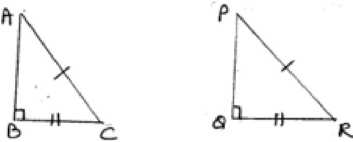Chapter – 7 Triangles

1. Congruence of Triangles
2. Criteria for Congruence of Triangles
3. Some Properties of a Triangle
4. Inequalities in a Triangle
• Triangle- A closed figure formed by three intersecting lines is called a triangle. A triangle has three sides, three angles and three vertices.
• Congruent figures- Congruent means equal in all respects or figures whose shapes and sizes are both the same for example, two circles of the same radii are congruent. Also two squares of the same sides are congruent.
• Congruent Triangles- two triangles are congruent if and only if one of them can be made to superpose on the other, so as to cover it exactly.

• If two triangles ABC and PQR are congruent under the correspondence A « P, B « Q and C « R then symbolically, it is expressed as DABC @ DPQR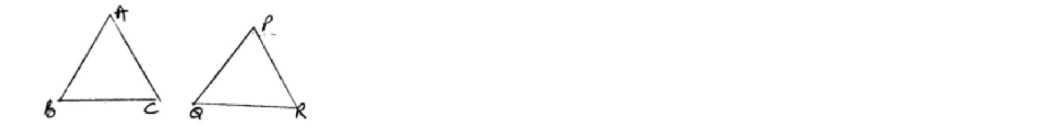• In congruent triangles corresponding parts are equal and we write ‘CPCT’ for corresponding parts of congruent triangles.

• SAS congruency rule – Two triangles are congruent if two sides and the included angle of one triangle are equal to the two sides and the included angle of the other triangle. For example: DABC and DPQR as shown in the figure satisfy SAS congruent criterion.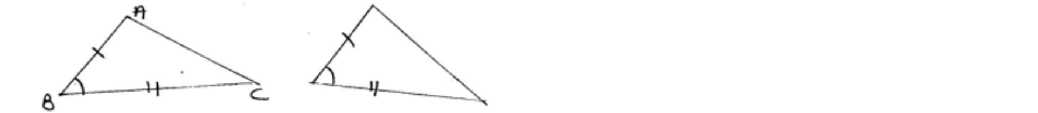• ASA Congruence Rule- Two triangles are congruent if two angles and the included side of

one triangle are equal to two angles and the included side of other triangle. For examples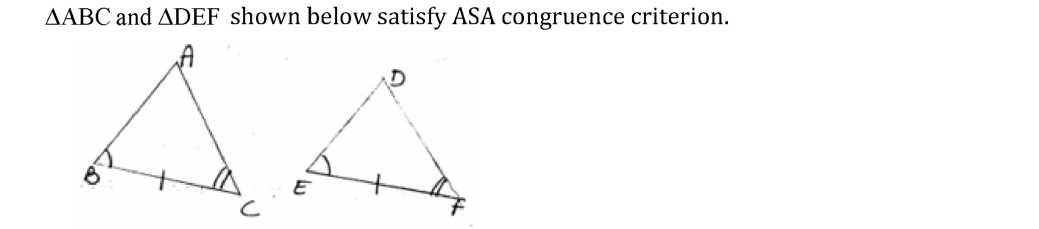• AAS Congruence Rule- Two triangle are congruent if any two pairs of angles and one pair of corresponding sides are equal for example DABC and DDEF shown below satisfy AAS
• Angle opposite to equal sides of a triangle are equal.

congruence criterion.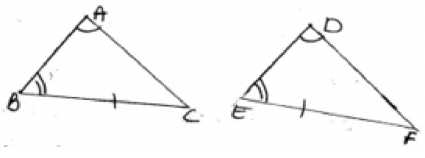• AAS criterion for congruence of triangles is a particular case of ASA criterion.
• Isosceles Triangle- A triangle in which two sides are equal is called an isosceles triangle.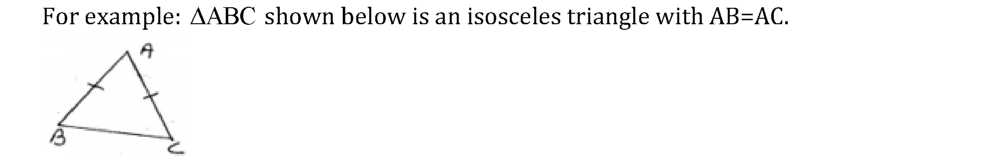• Sides opposite to equal angles of a triangle are equal.
• Each angle of an equilateral triangle is 60o.
• SSS congruence Rule – If three sides of one triangle are equal to the three sides of another triangle then the two triangles are congruent for example DABC and DDEF as shown in

the figure satisfy SSS congruence criterion.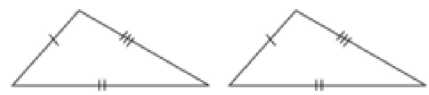• RHS Congruence Rule- If in two right triangles the hypotenuse and one side of one triangle are equal to the hypotenuse and one side of the other triangle then the two triangle are congruent. For example: DABCand DPQR shown below satisfy RHS

congruence criterion.RHS stands for right angle – Hypotenuse side.

• A point equidistant from two given points lies on the perpendicular bisector of the line segment joining the two points and its converse.
• A point equidistant from two intersecting lines lies on the bisectors of the angles formed by the two lines.
• In a triangle, angle opposite to the longer side is larger (greater)
• In a triangle, side opposite to the large (greater) angle is longer.
• Sum of any two sides of a triangle is greater than the third side.
Previous articleChapter 6 Lines and Angles Notes for Class 9th Maths